### Dice 3

The figure given on the left hand side in each of the following questions is folded to form a box. Choose from the alternatives (1), (2), (3) and (4) the boxes that is similar to the box formed.

1.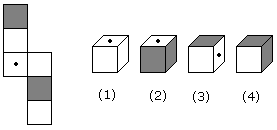A. 2 and 3 only B. 1, 3 and 4 only C. 2 and 4 only D. 1 and 4 only
2.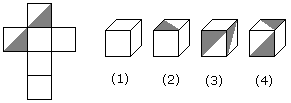A. 1, 2 and 4 only B. 3 and 4 only C. 1 and 2 only D. 1, 2 and 3 only
3.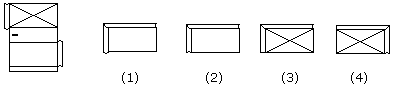A. 1 only B. 2 only C. 3 only D. 4 only
4.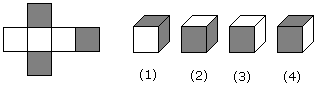A. 1 and 3 only B. 2 and 4 only C. 2 and 3 only D. 1, 2, 3 and 4
5.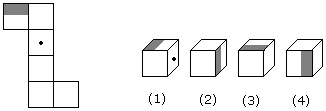A. 1 and 2 only B. 2 and 3 only C. 1, 2, 3 and 4 D. 2 and 4 only
6.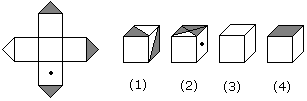A. 1 and 2 only B. 2 and 4 only C. 2 and 3 only D. 1 and 4 only